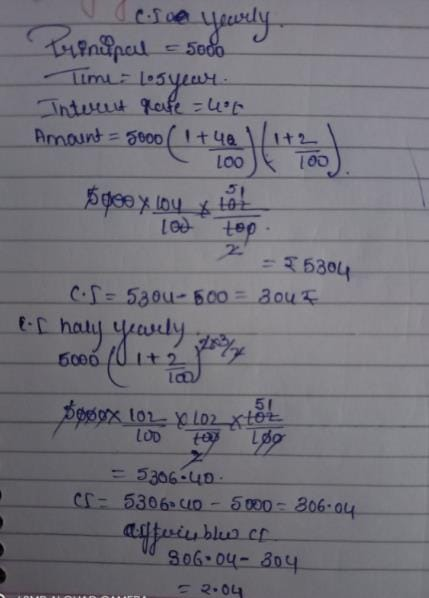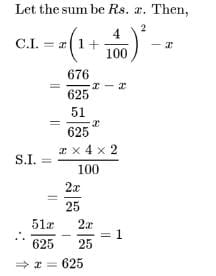Test: Compound Interest- 1

# Test: Compound Interest- 1

Test Description

## 10 Questions MCQ Test CSAT Preparation for UPSC CSE | Test: Compound Interest- 1

Test: Compound Interest- 1 for Banking Exams 2023 is part of CSAT Preparation for UPSC CSE preparation. The Test: Compound Interest- 1 questions and answers have been prepared according to the Banking Exams exam syllabus.The Test: Compound Interest- 1 MCQs are made for Banking Exams 2023 Exam. Find important definitions, questions, notes, meanings, examples, exercises, MCQs and online tests for Test: Compound Interest- 1 below.
Solutions of Test: Compound Interest- 1 questions in English are available as part of our CSAT Preparation for UPSC CSE for Banking Exams & Test: Compound Interest- 1 solutions in Hindi for CSAT Preparation for UPSC CSE course. Download more important topics, notes, lectures and mock test series for Banking Exams Exam by signing up for free. Attempt Test: Compound Interest- 1 | 10 questions in 10 minutes | Mock test for Banking Exams preparation | Free important questions MCQ to study CSAT Preparation for UPSC CSE for Banking Exams Exam | Download free PDF with solutions
 1 Crore+ students have signed up on EduRev. Have you?
Test: Compound Interest- 1 - Question 1

### A bank offers 5% compound interest calculated on half-yearly basis. A customer deposits Rs. 1600 each on 1st January and 1st July of a year. At the end of the year, the amount he would have gained by way of interest is:

Test: Compound Interest- 1 - Question 2

### What will be the compound interest on a sum of Rs. 40,000 after 3 years at the rate of 11 p.c.p.a.?

Detailed Solution for Test: Compound Interest- 1 - Question 2

Amount after 3 years = P(1 + R/100)T
=> 40000(1 + 11/100)3
=> 40000(111/100)3
=> 40000[(111*111*111)/(100*100*100)]
=> (4*111*111*111)/100
=> 54705.24
Compound Interest = 54705.24 - 40000
= Rs. 14705.24

Test: Compound Interest- 1 - Question 3

### Q. What is the difference between the compound interests on Rs. 5000 for 11/2  years at 4 percent per annum compounded yearly and half yearly?

Detailed Solution for Test: Compound Interest- 1 - Question 3Test: Compound Interest- 1 - Question 4

If the simple interest on a sum of money for 2 years at 5% per annum is Rs. 60, what is the compound interest on the same at the same rate and for the same time?

Test: Compound Interest- 1 - Question 5

The difference between compound interest and simple interest on an amount of Rs. 15,000 for 2 years is Rs. 96. What is the rate of interest per annum?

Detailed Solution for Test: Compound Interest- 1 - Question 5

[15000×(1+R/100)2 − 15000] - (15000×R×2)/100 = 96
⇒15000[(1+R/100)2 − 1 − 2R/100]=96
⇒ 15000[(100+R)2 − 10000−(200×R)]/10000 = 96
⇒ R2 = (96×23) = 64
⇒ R = 8
∴ Rate = 8%

Test: Compound Interest- 1 - Question 6

The compound interest on Rs. 30,000 at 7% per annum is Rs. 4347. The period (in years) is:

Detailed Solution for Test: Compound Interest- 1 - Question 6

Amount = Rs.(30000+4347) = Rs.34347
let the time be n years
Then,30000(1+7/100)n = 34347
(107/100)n = 34347/30000
= 11449/10000
= (107/100)2
n = 2years

Test: Compound Interest- 1 - Question 7

At what rate of compound interest per annum will a sum of Rs. 1400 become Rs. 1573.04 in 2 years?

Detailed Solution for Test: Compound Interest- 1 - Question 7

Let the rate be R% per annum
P(1+R/100)T = 1573.04
= 1400(1+R/100)2 = 1573.04
= (1+R/100)2 = 1573.04/1400 = 157304/140000 = 11236/10000
(1+R/100) = (√11236/10000)
= (√11236)/(√10000)
= 106/100
R/100 = (106/100 − 1)
=> 6/100
R = 6%

Test: Compound Interest- 1 - Question 8

There is 80% increase in an amount in 8 years at simple interest. What will be the compound interest of Rs. 14,000 after 3 years at the same rate?

Detailed Solution for Test: Compound Interest- 1 - Question 8

Simple interest = P * r * t /100, where, P is the Principal, r is the rate of interest and t is the time period such that the rate of interest and the time period have mutually compatible units like r % per annum and t years.

Here, in the first case, let the Principal be Rs.P and rate of interest be r % per annum. Time period is given as 8 years . Interest is given as 80 P / 100
So, 80 P / 100 = P * r * 8 / 100
Or, 80 = 8 r
So, r = 10 % per annum.

Now, in the second case, Principal is given as Rs. 14,000, rate of compound interest is 10 % per annum as determined above; and t is 3 years.
Compound interest = P * ( 1 + r/100)t - P
= 14,000 * ( 1+ 10 /100)3 - 14,000
= 14,000 * ( 1 + 0.1)3 - 14,000
= 14,000 *(1.1)3 - 14,000
(14,000 * 1.331) - 14,000
= 18,634 - 14,000 = 4,634
So, the compound interest on Rs.14,000 at the same rate of interest as in case 1 in 3 years will be Rs. 4,634.

Test: Compound Interest- 1 - Question 9

The difference between simple and compound interests compounded annually on a certain sum of money for 2 years at 4% per annum is Re. 1. The sum is:

Detailed Solution for Test: Compound Interest- 1 - Question 9Test: Compound Interest- 1 - Question 10

The difference between simple interest and compound on Rs. 900 for one year at 10% per annum reckoned half-yearly is:

## CSAT Preparation for UPSC CSE

72 videos|64 docs|92 tests
 Use Code STAYHOME200 and get INR 200 additional OFF Use Coupon Code
Information about Test: Compound Interest- 1 Page
In this test you can find the Exam questions for Test: Compound Interest- 1 solved & explained in the simplest way possible. Besides giving Questions and answers for Test: Compound Interest- 1, EduRev gives you an ample number of Online tests for practice

## CSAT Preparation for UPSC CSE

72 videos|64 docs|92 tests